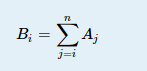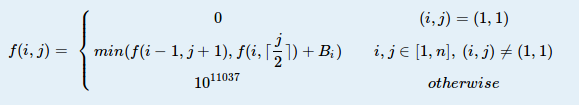odd doctor 已更新

# MZL's munhaff function

Time Limit
3s
Memory Limit
131072KB
Judge Program
Standard
Ratio(Solve/Submit)
50.00%(1/2)
Description:

MZL is a mysterious mathematician, and he proposed a mysterious function at his young age.
First of all, given n positive integers Ai and AiAi+1.

Then, generate n positive integers BiDefine f(i,j) for i,jZFind f(n,1).

Input:

The first line of the input contains a single number T, the number of test cases.
For each test case, the first line contains a positive integer n, and the next line contains n positive integers Ai.
T100, 1n105, n106, 1Ai104.

Output:

For each test case, output f(n,1) in a line.

Sample Input:
3
3
1 1 1
5
28 26 25 24 1
10
996 901 413 331 259 241 226 209 139 49
Sample Output:
5
233
11037
Hint:

case 1 ：f(1,1)=0f(1,2)=f(1,1)+3=3f(1,3)=f(1,2)+3=6f(2,1)=min(f(2,1)+2,f(1,2))=3f(2,2)=min(f(2,1)+2,f(1,3))=5f(2,3)=f(2,2)+2=7f(3,1)=min(f(3,1)+1,f(2,2))=5

Source:

2015 Multi-University Training Contest 5

Submit